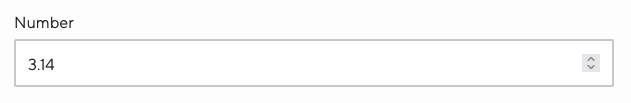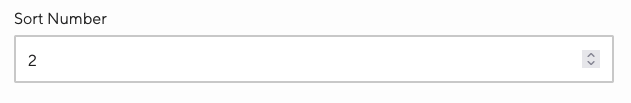# Using Number Inputs to edit your data

Working with a specific static site generator?
Customize CloudCannon's documentation to suit your SSG.

Great! We'll show you documentation relevant to .
You can change this any time using the dropdown in the navigation bar.

These inputs are for editing numerical values in your data.

## Number#

Single line input field for integers or decimals.

Number inputs are shown for inputs configured with the type `number`, or input keys matching:

• `number`
• `*_number`Naming convention
copied
Naming convention
copied
Naming convention
copied
Input configuration
copied
Input configuration
copied
Input configuration
copied

## Range#

A combination number and range input field for integers or decimals.

Range inputs are shown for inputs configured with the type `range`. This input requires `step`, `min` and `max` options.Input configuration
copied
Input configuration
copied
Input configuration
copied

## Options#

Number input options are configured with `options` inside an `_inputs` entry.

`min` - Float#

The lowest value in the range of permitted values.

`max` - Float#

The greatest value in the range of permitted values.

`step` - Float or "any"#

A number that specifies the granularity that the value must adhere to, or the special value `any`, which allows any decimal value between `max` and `min`.

`empty_type` - string#

Set how an 'empty' value will be saved. Does not apply to existing empty values. Can be one of the following:

• `number` - an empty value for this input will be stored as `0` (default).
• `null` - an empty value for this input will be stored as a null value. This does not apply to TOML files.

## Valid values#

Documented below are the valid formats for number and range inputs.

The lists of examples are non-exhaustive.

Binary and hexadecimal numbers will be converted to base-10 integers when loaded into the Data Editor

Empty/null value:

• `number: `

Whole base-10 integers (unquoted):

• `number: 123456`
• `number: -123456`
• `number: 123456e78`
• `number: 123456e+78`
• `number: 123456e-78`

Floating point numbers (unquoted):

• `number: 123.456`
• `number: -123.456`
• `number: 123.456e78`
• `number: 123.456e+78`
• `number: 123.456e-78`

Binary integers (unquoted):

• `number: 0b11110001001000000`

• `number: 0x1E240`

Whole base-10 integers (unquoted):

• `number = 12346`
• `number = +123456`
• `number = -123456`
• `number = 123_456`

Floating point numbers (unquoted):

• `number = 123.456`
• `number = +123.456`
• `number = -123.456`
• `number = 123.456e78`
• `number = 123.456E78`
• `number = 123.456e09`
• `number = 123.456e+78`
• `number = 123.456e-78`
• `number = -123.456e78`
• `number = 123_456.789_012`

• `number = 0x1E240`

Octal numbers (unquoted):

• `number = 0o361100`

• `number = 0b11110001001000000`

Null value:

• `"number": null`

Base-10 integers (unquoted):

• `"number": 123456`
• `"number": -123456`
• `"number": 123456e78`
• `"number": -123456e78`

Floating-point numbers (unquoted):

• `"number": 123.456`
• `"number": -123.456`
• `"number": 123.456e78`
• `"number": 123.456E78`
• `"number": 123.456e+78`
• `"number": -123.456e78`